## Example Questions

### Example Question #1 : Understanding Functions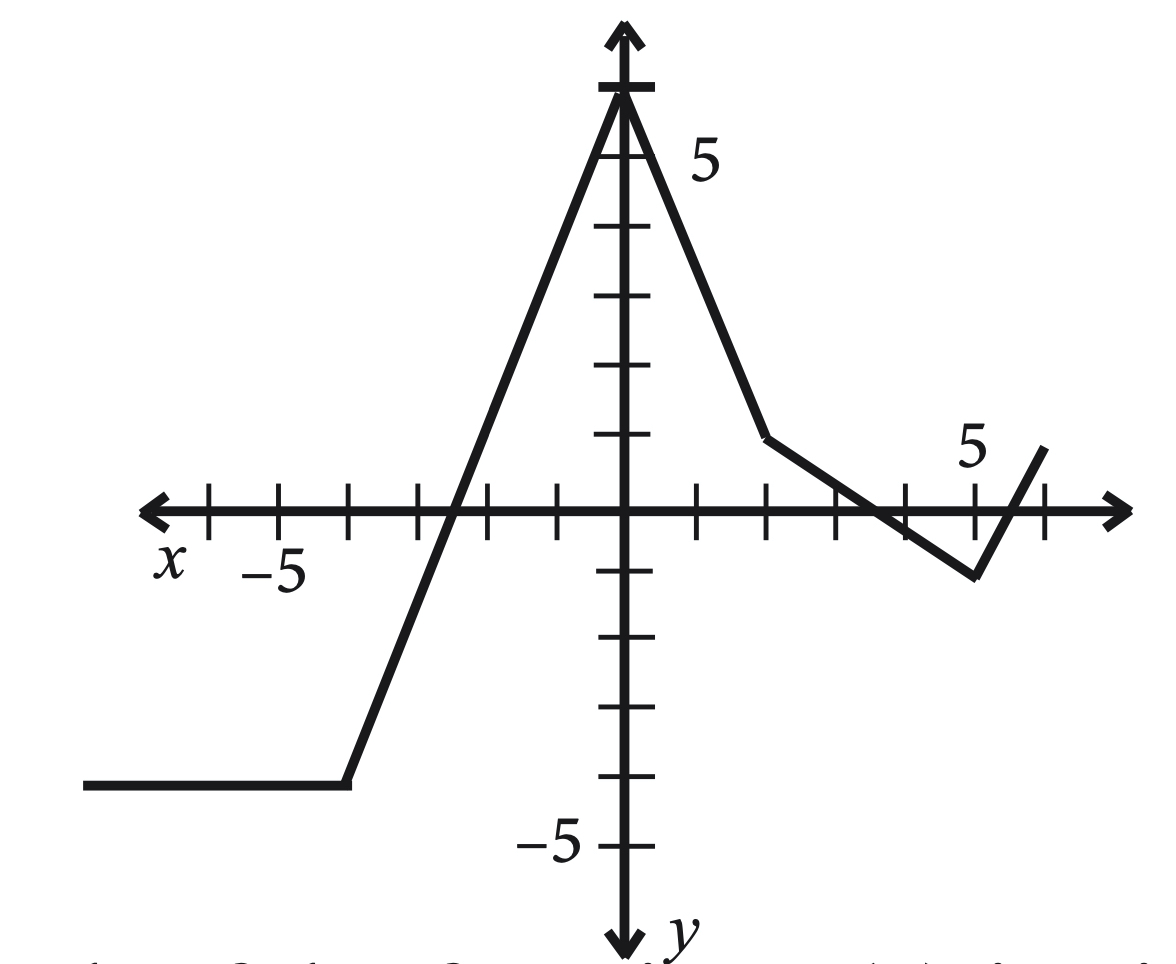The graph of the function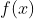is given above for all numbers within its domain. At what value of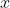is the function at its maximum?

-4

7

0

6

0

Explanation:

Some SAT questions ask you to interpret the graph of a function, even if you are not given the algebraic notation for that function. For these, recognize that when a function is graphed, the value of the function is shown on the y-axis (the vertical height) and the input values are displayed on the x-axis (the horizontal). So when this question asks you for the maximum of the function, look for the highest spot on the graph. That takes place at the point (0, 6). Since the question asks for the x-value at which the function is maximized, you'll then use x = 0 as your answer.

### Example Question #1 : Understanding Functions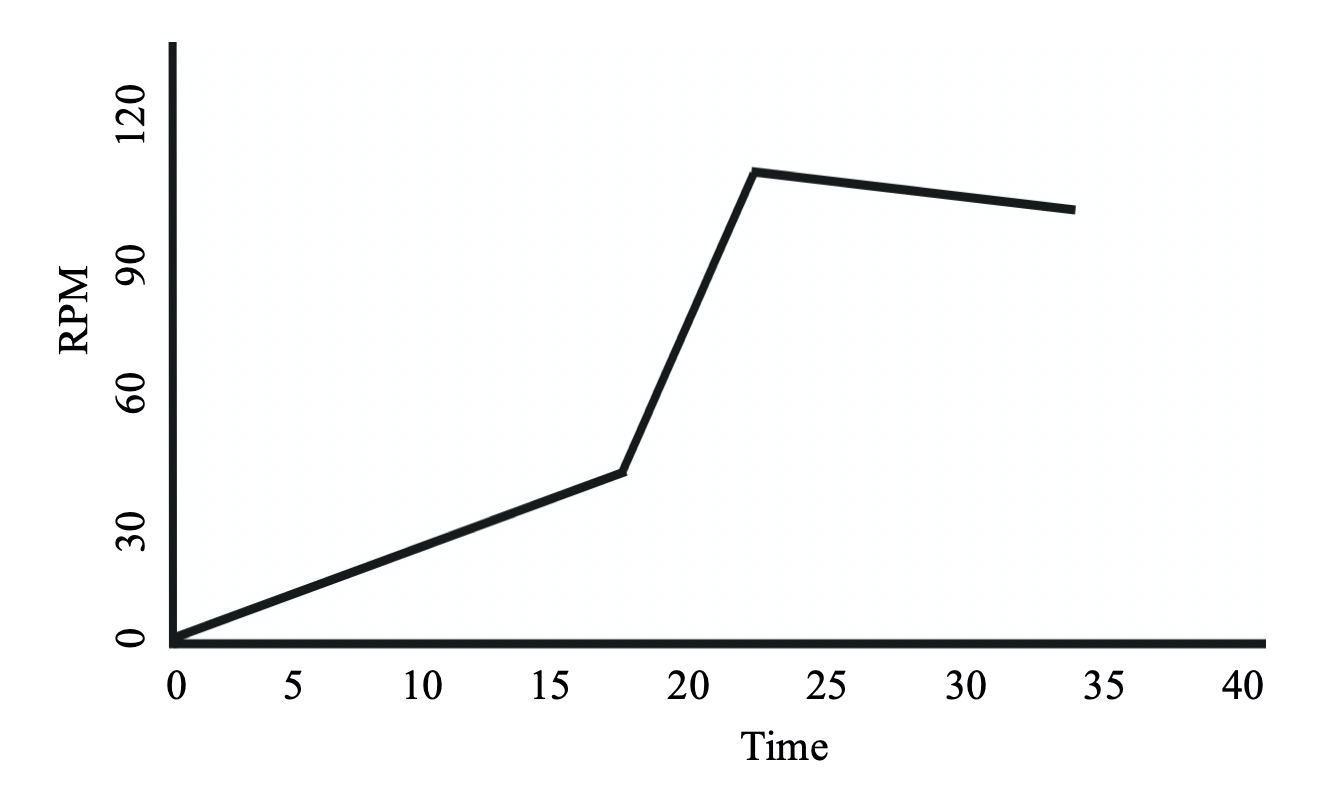The graph above plots Jessica's workout in a 35-minute spin class, with her number of pedal revolutions per minute (RPM) graphed as a function of elapsed time. Approximately how many minutes into the workout was her revolutions per minute the highest?

21

35

18

29

21

Explanation:

This problem asks you to interpret the graph of a function. As you can see, revolutions per minute are graphed on the y-axis, so to find her highest RPM you're looking for the highest point on the graph. But the question asks for how many minutes she was into the workout at that point, and minutes are on the x-axis. So when you find her highest point, then scan down to the x-axis to see the time, which is at approximately 21 minutes.

### Example Question #1 : Understanding Functions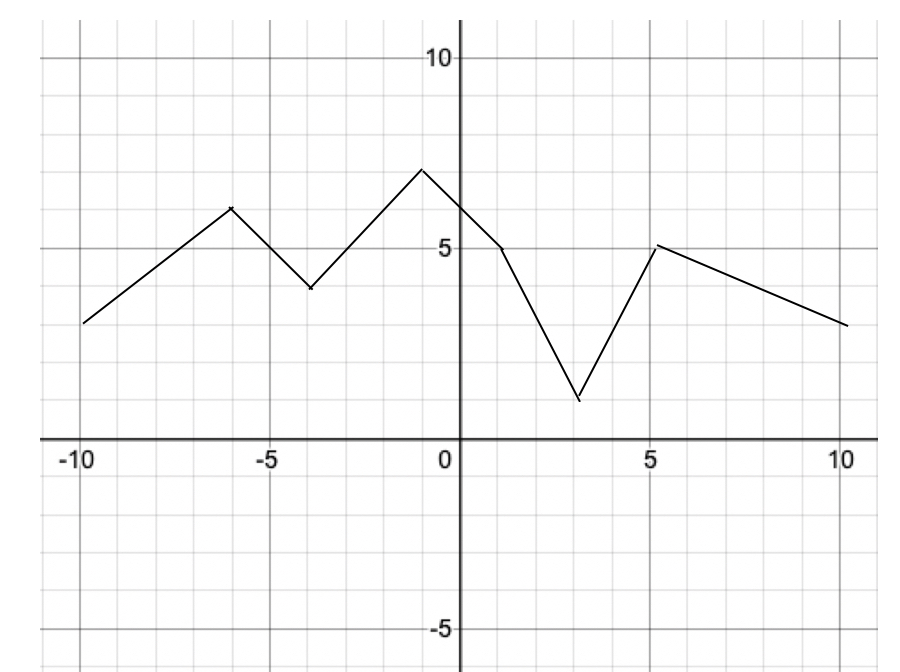The graph of the functionis shown above for all numbers within its domain. What is the difference between the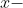coordinates at the points at which the function is at its minimum and at its maximum?

20

4

6

9

4

Explanation:

When approaching this problem, it is important to know that in functions,, graphed on the y-axis, is the value of the function, the output value. Andis the input value. So when you're asked to find the minimum and maximum of a function, you're looking on the y-axis for the lowest (minimum) and highest (maximum) points. Those points here occur at (-1, 7) for the maximum (that's where the function has a value of 7) and at (3, 1) for the minimum (that's where the function has a value of 1). Since the question asks for the difference in thecoordinates at those points, you'll take those coordinates of -1 and 3 and. The difference is then 3 - (-1) = 4.

### Example Question #2 : Understanding Functions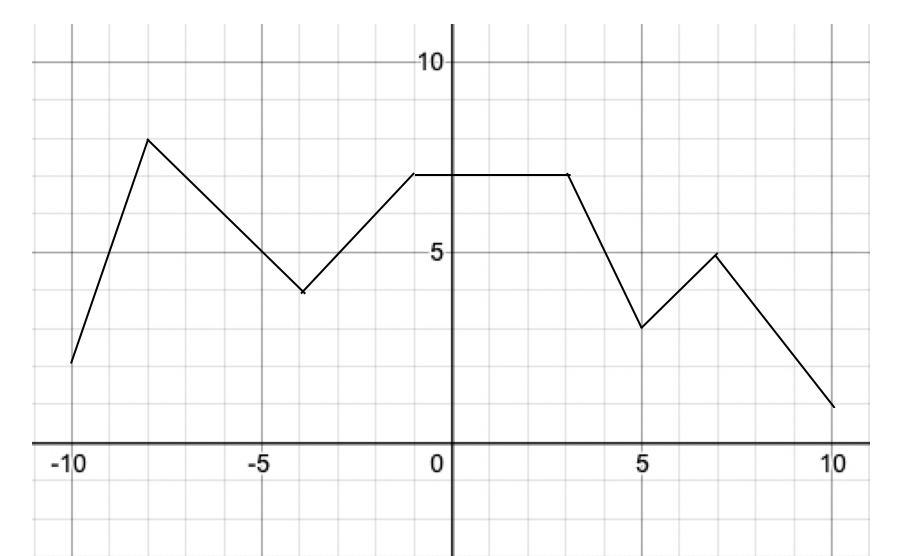The graph of the function f(x) is given above for all numbers within its domain. At what value of x is the function at its minimum?

-4

2

10

-10

10

Explanation:

An important thing to know about functions is that f(x) is defined as the "function" itself, where x is an input value that goes into the function. So when you're asked for where a function is at its minimum, you're looking on the y-axis for the lowest point.  Here that point is at (10, 1). The question asks for x value, so the correct answer is 10.

### Example Question #3 : Understanding Functions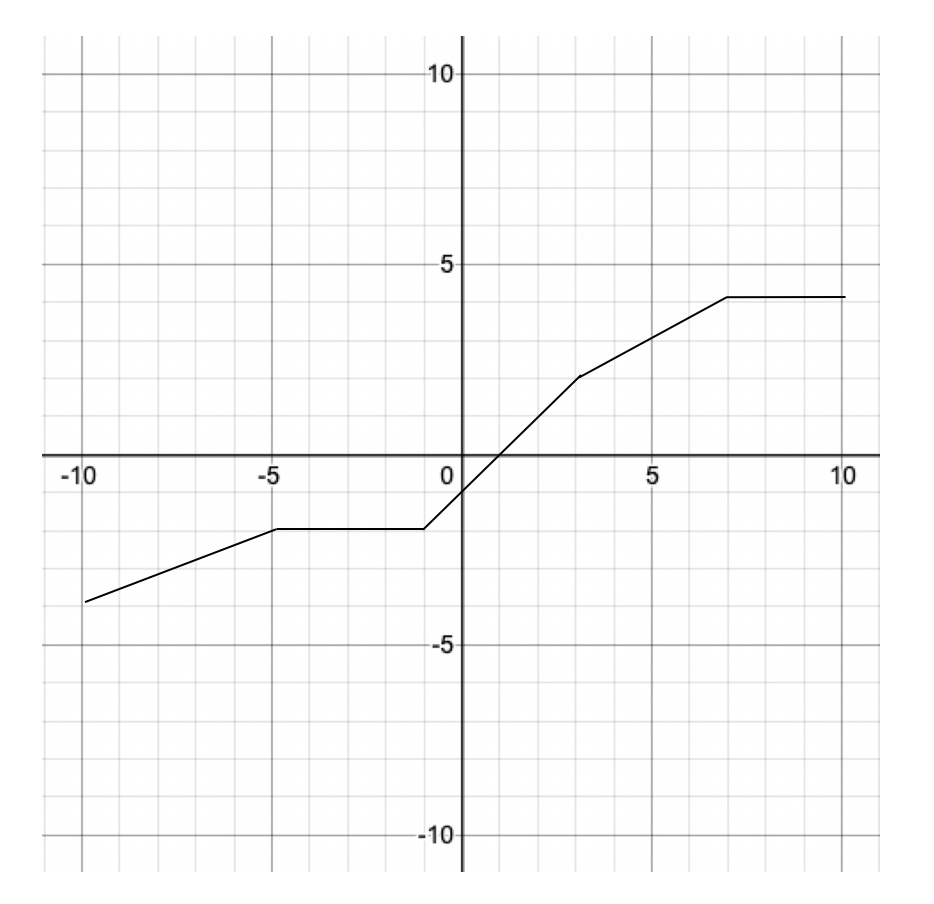The graph of the function g(x) is given above for all numbers within its domain. At what value of x does the function equal zero?

2

-1

1

0

1

Explanation:

An important consideration when you're interpreting graphs of functions is that the value of the function itself - in this case the function g(x) - is marked on the y-axis (the vertical axis). The x-value is an input value to the function, and the y-value is the value of the function itself. So here you're looking for where the graph crosses the x-axis, at which point y=0.  This happens at the point (1, 0). And since the question asks you for the value of x at this point, you'll then provide the correct answer, x = 1.

### Example Question #6 : Understanding Functions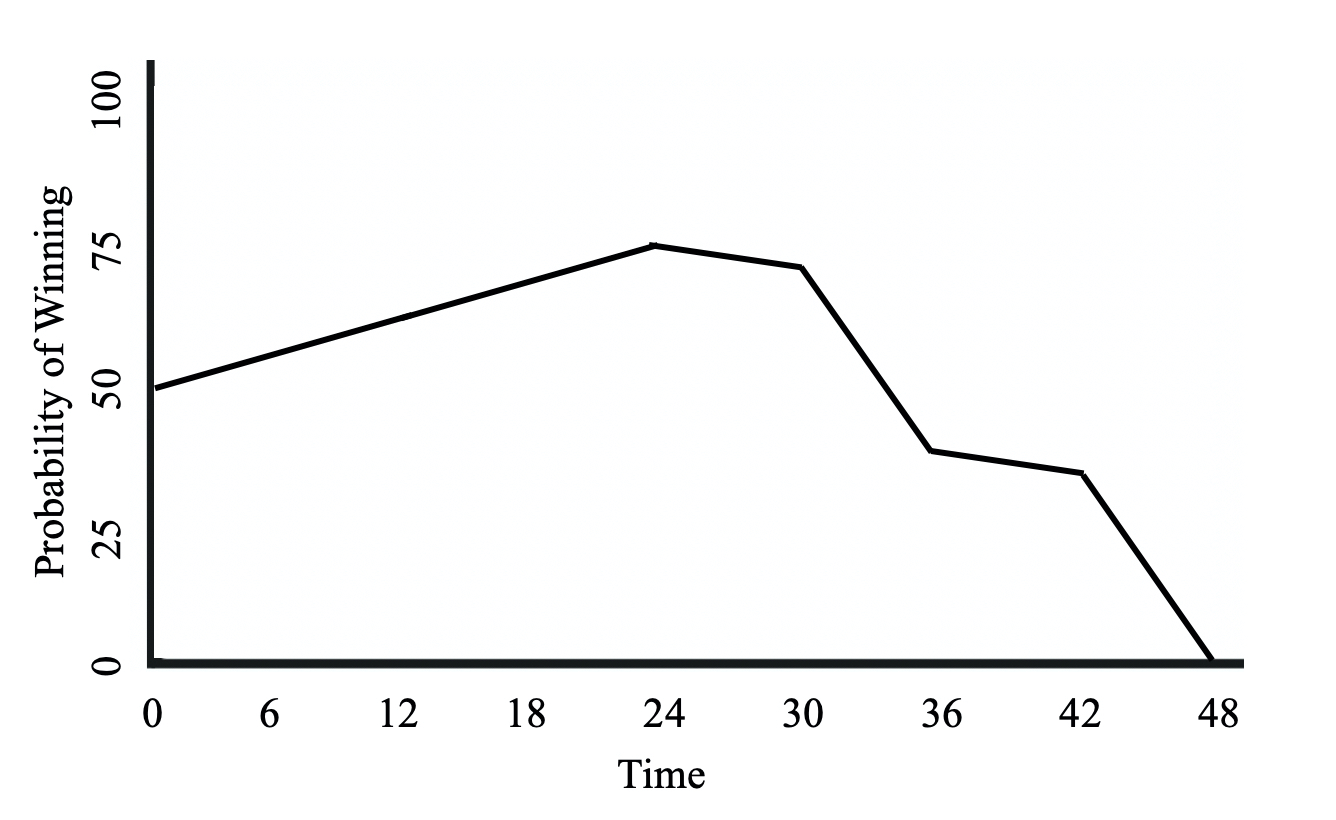The graph above is a function of a basketball team's probability of winning (shown on the y-axis) as a function of the time elapsed during a 48-minute game (shown on the x-axis). Which of the following best describes the team's performance during the game?

The team never had a lead during the second half of the game.

The team played better in the second half of the game than it did during the first.

The team was significantly better than its opponent during the first half of the game.

The team lost the game.

The team lost the game.

Explanation:

As you can see from the graph, the team gradually increased its probability of winning during the first half of the game, then that probability began to drop gradually in the second half, with two sharp drops until the probability reached 0.  This demonstrates that the team lost the game - at the end, its probability of winning was zero. While the team did increase its probability of winning throughout the first half, it did so gradually, so the idea that it was "significantly better than its opponent" is not necessarily true.

### Example Question #4 : Understanding Functions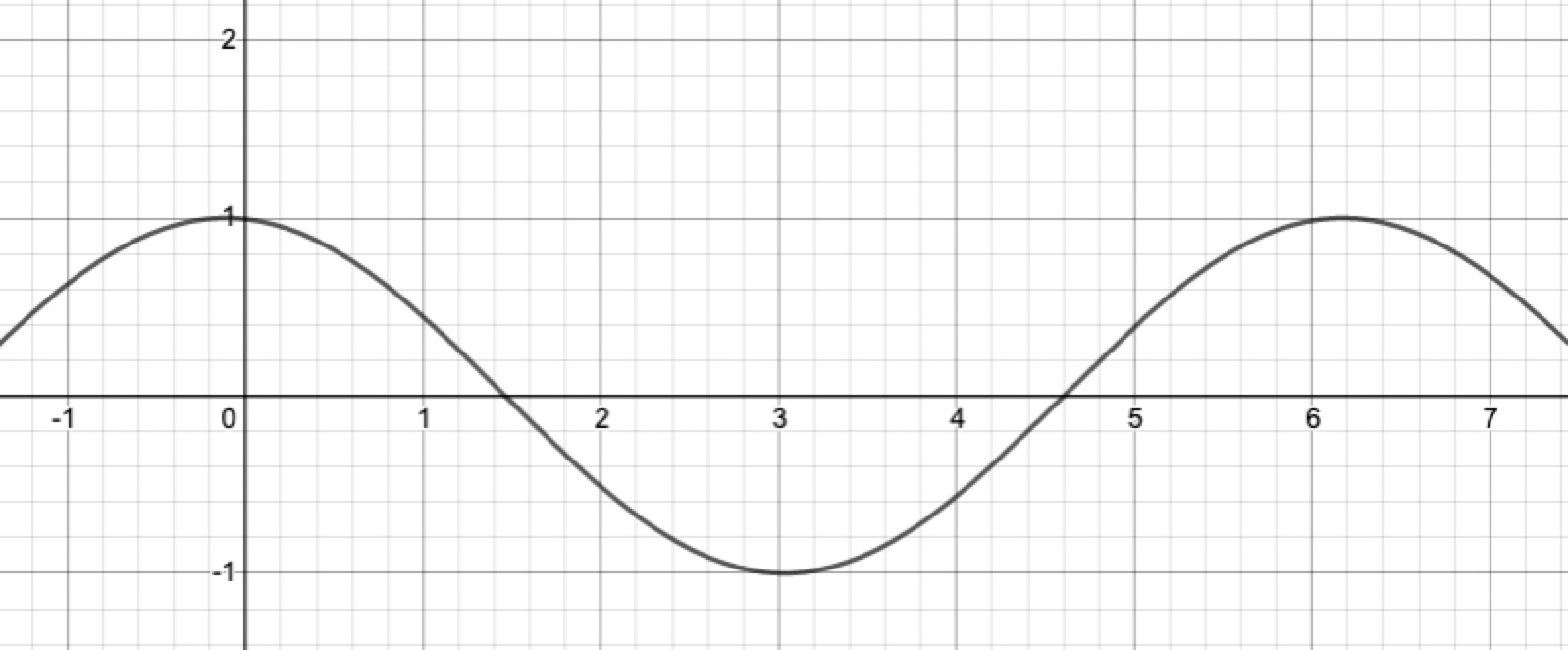The graph of the function f(x) is given above for all numbers within its domain. At approximately what value of x is the function at its minimum?

3

1

-1

6

3

Explanation:

Importantly when you are dealing with functions, you should know that the value of the function itself is shown on the y-axis (the vertical axis) and the x-axis shows you the input value of x that leads to the value of the function.  So here when you're asked for the minimum value of the function, you should look for the lowest point on the graph.  That appears at approximately (3, -1).  The question asks for the x-value at that point, so the correct answer is 3.

### Example Question #5 : Understanding Functions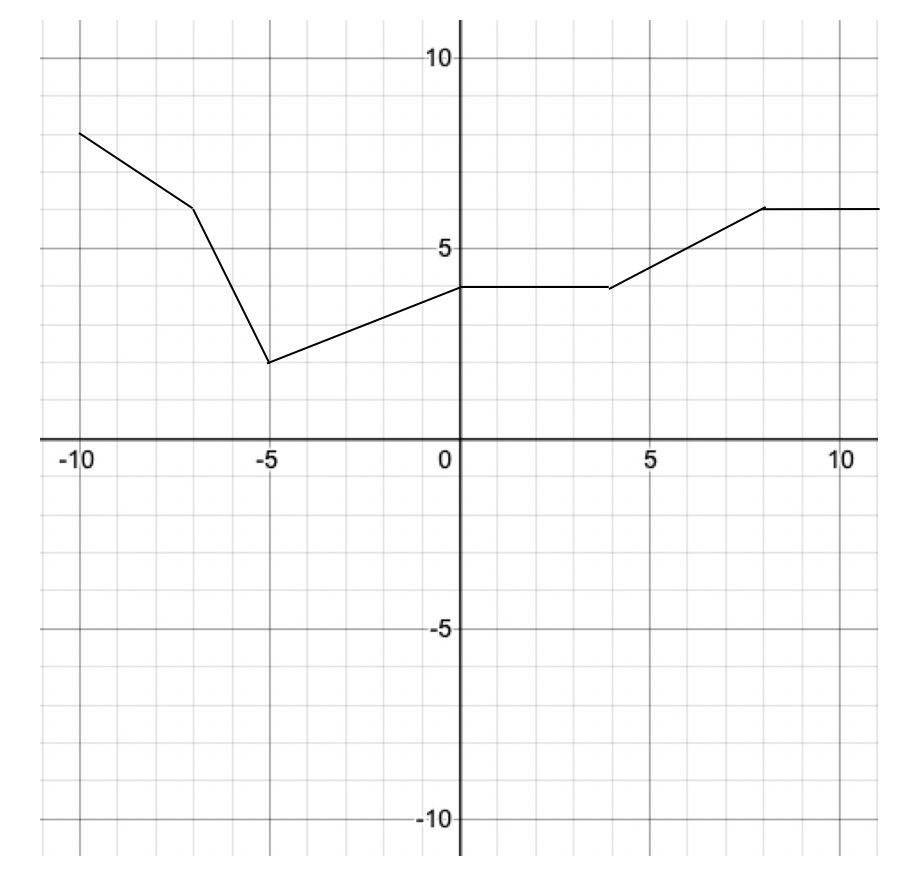The function f(x) is shown above for all the numbers within its domain. What is the value of the function when it is at its minimum?

-5

0

4

2

2

Explanation:

When you're interpreting graphed functions, it is important to recognize that the value of the function itself is shown on the y-axis (the vertical axis) while the horizontal axis displays the x-values that are inputs to the function. So here when you're asked for the minimum value of the function, you're looking for the lowest point, which happens at the point (-5, 2). And since the value of the function is the y-coordinate at that point, you can answer 2 as the correct answer.

### Example Question #6 : Understanding Functions

The polynomial functionhas zeroes at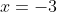and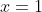. Which of the following could be the graph of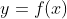in the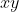plane?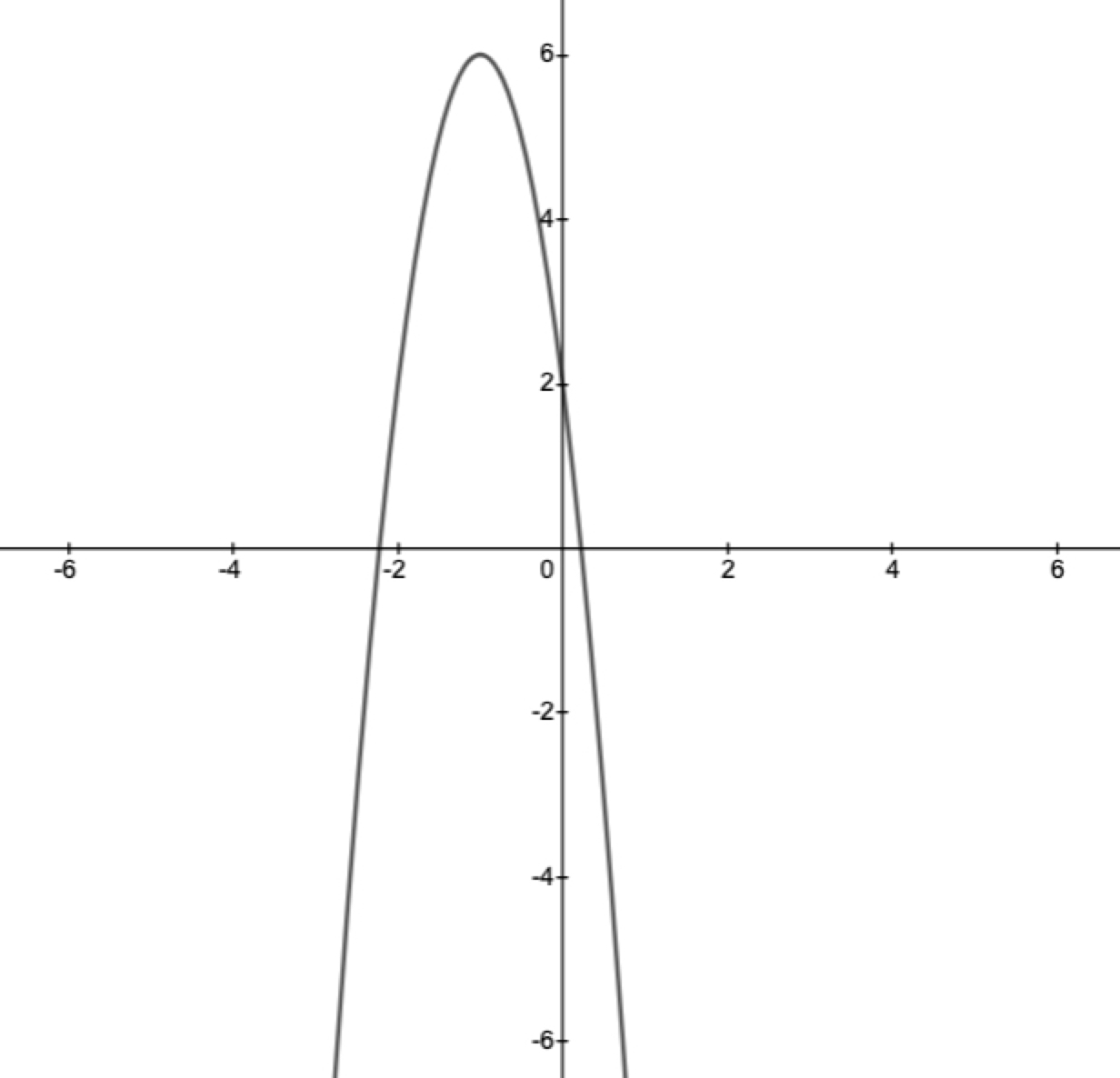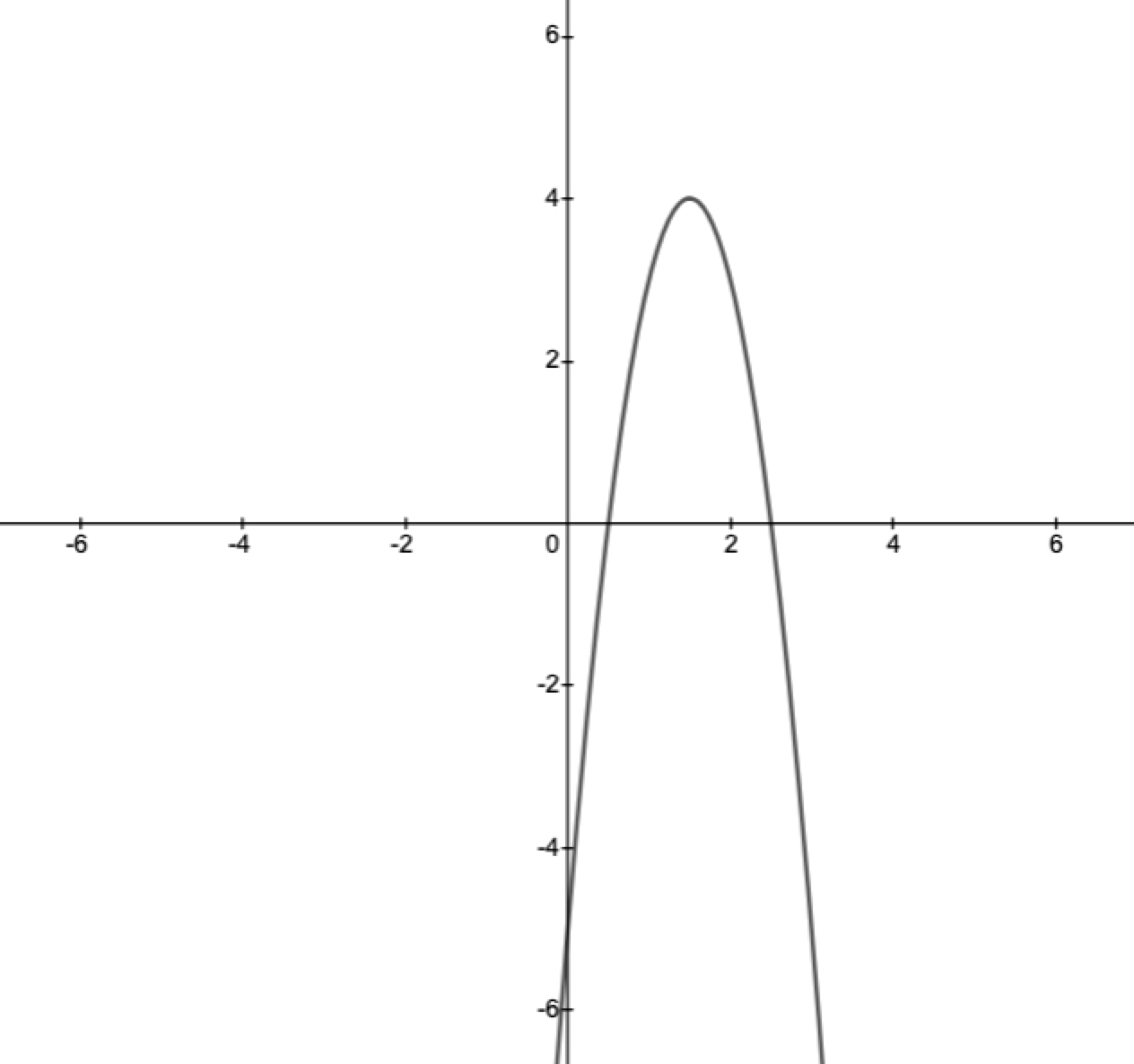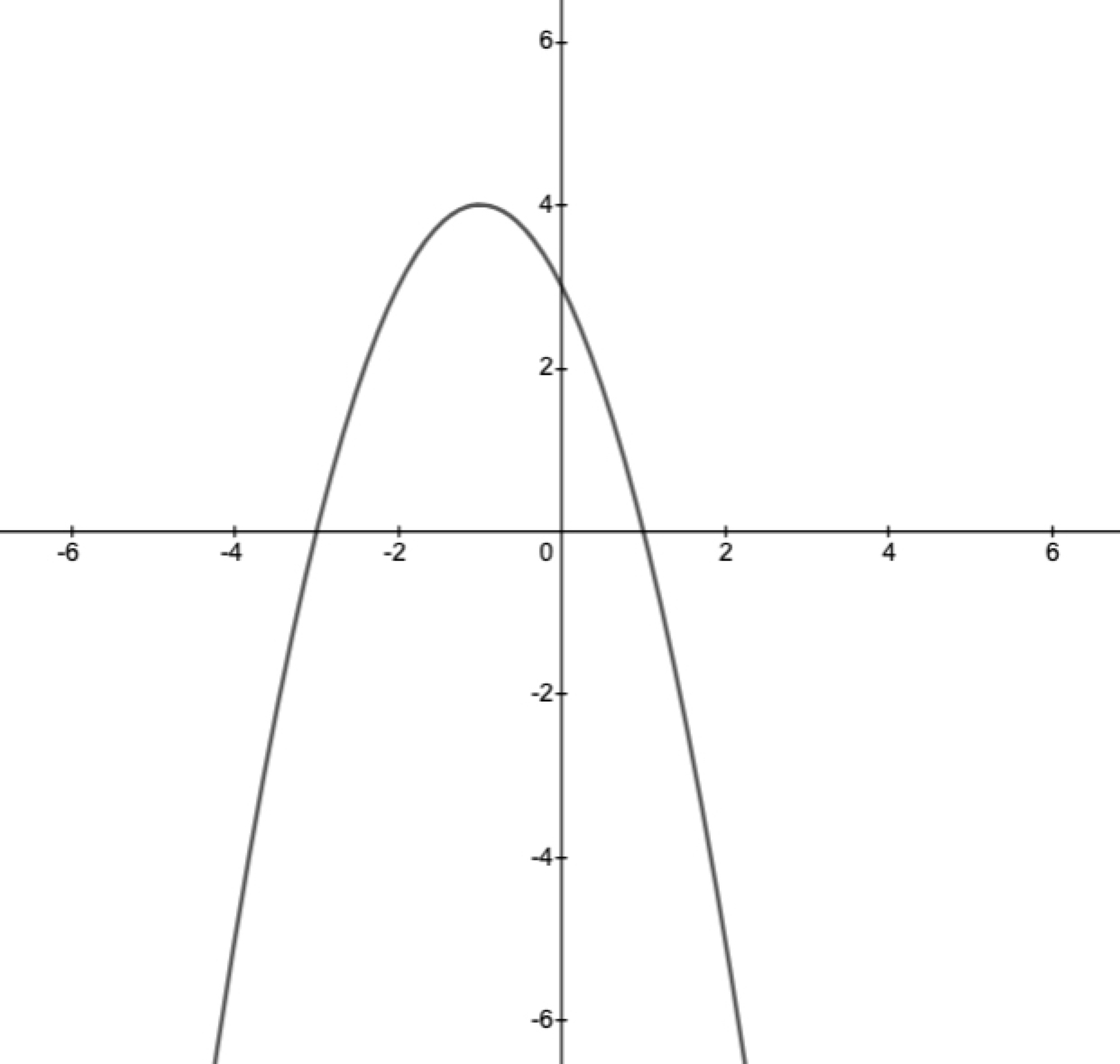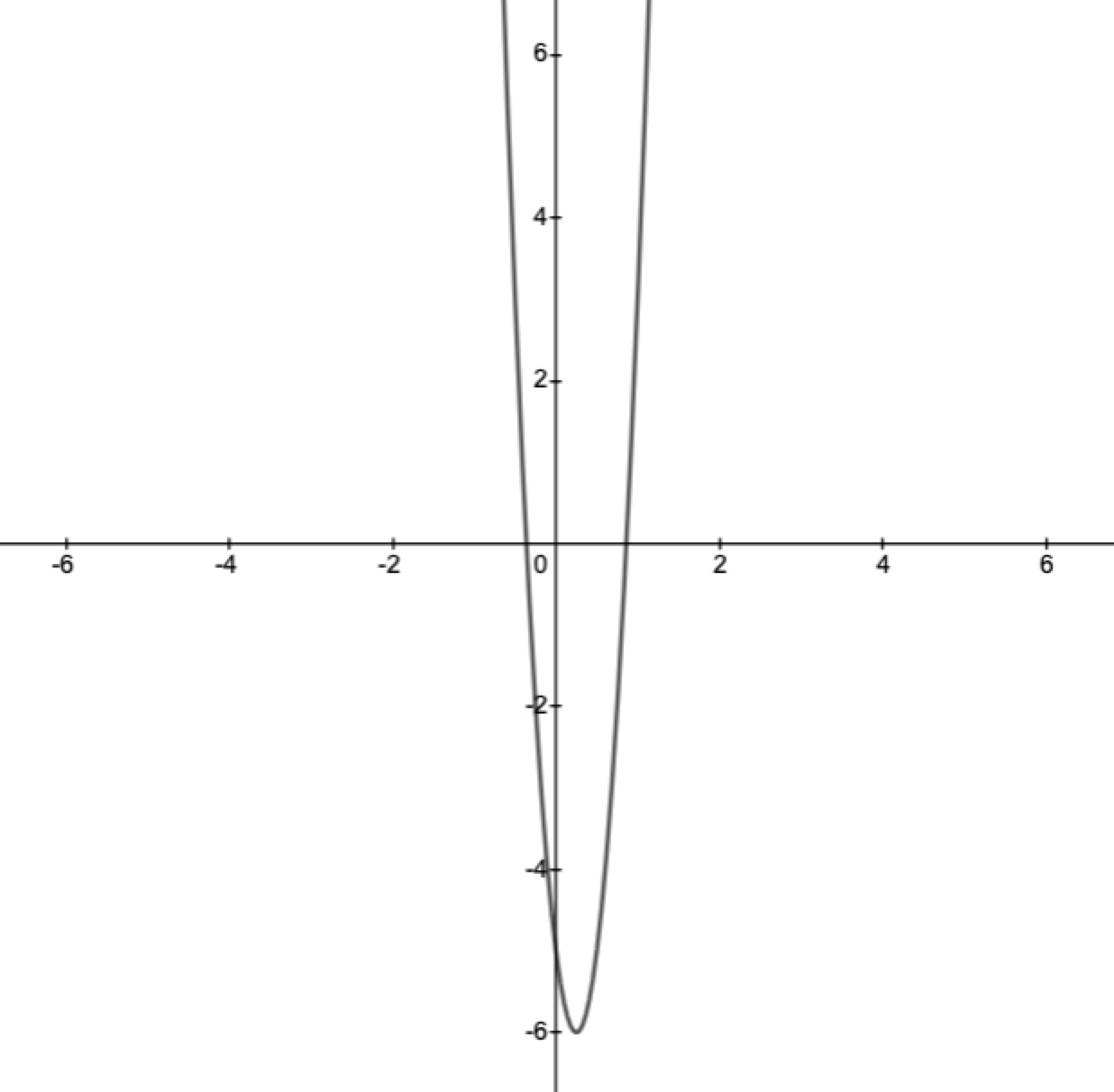Explanation:

On a problem such as this that asks you to match a graph with a function, you need to use process of elimination using the characteristics of the function that you were provided. Here you're told where the zeroes are, meaning that at those points,and, the graph should cross the x-axis. Only one graph meets those criteria, so you have your correct answer.

### Example Question #2 : Understanding Functions

If n is a constant in the function f(x) = nx + 5, and f(14) = -2, what is f(2)?

4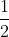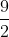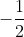4

Explanation:

In this instance, we can use the outcome of the function when our input is 14 to solve for “n” and thus, understand our full function. If

f(14) = -2 and f(x) = nx + 5, then

n(14) + 5 = -2

If we isolate n, we arrive at

n(14) = -7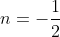Be careful… we can’t stop here! The question hasn’t asked us to solve for n, it has asked us for f(2). So, if we know that our function, with n as a known constant, is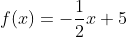, then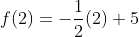, or 4.

### All SAT Mathematics Resources